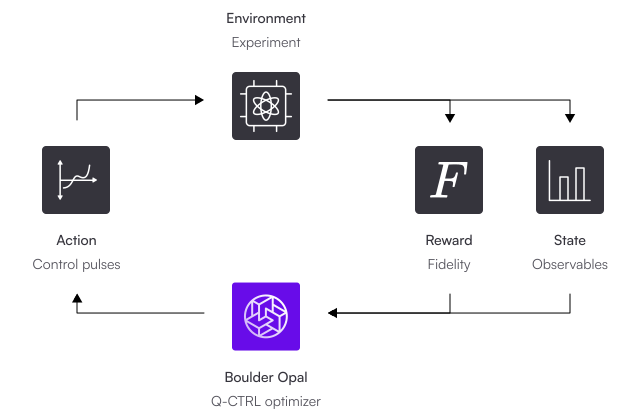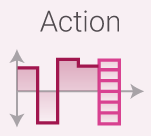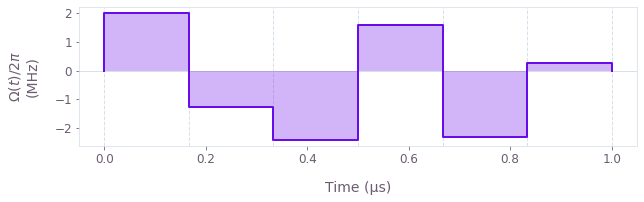•Boulder Opal
• User guides
• Optimize controls starting from an incomplete system model

# How to optimize controls starting from an incomplete system model

Design waveforms using a model-independent reinforcement learning framework

Boulder Opal contains reinforcement learning (RL) tools that allow you to generate optimized controls without requiring a complete understanding of the model of your quantum system. Here we will show you how to apply policy gradient reinforcement learning to generate novel control pulses with high fidelity. Pulses generated in this way have been used to improve the performance of single and two-qubit gates on IBM Quantum hardware.

When you use our RL tools, a so-called RL agent learns the relationship between control pulses and the device measurement by direct iterative interaction. The agent gains an implicit understanding of the device model from receiving measurement data after each segment of a control pulse. The learning cycle is illustrated in the figure below, where a fidelity-based reward is returned to the agent after each complete control pulse; in general you can also return a reward for every pulse segment (combining the two loops on the right).## Summary workflow

### 1. Set up the environment for your use case

The reinforcement learning framework consists of an agent that learns to maximize a reward signal by interacting with an environment and thereby exploring different sequences of actions. In a practical situation, this environment interaction occurs through the interface to your quantum hardware, and must include state preparation, manipulation, and measurement. The same interactions must be specified when the environment is a simulated quantum system, as in this guide.

To set up the environment, you need to establish the interface for applying the agent's actions to the device or simulation, as well as obtaining the measurements and reward associated with each action.

#### Action interface

The RL agent can take discrete actions in an action space with a size that you must specify. As such, you must first discretize the space of your available actions on the environment, and provide a mapping between actions and non-negative integer indices (the agent will return a non-negative integer to indicate the next action). Note that a single action index can be mapped to indicate particular values for multiple control variables.

This discretization is illustrated below for a pulse composed of piecewise-constant segments. In this case, the agent's action index indicates the specific value of the control segment to be applied to the environment.#### Measurements and reward

Since the learning agent requires feedback from the environment after each action to decide on the next, the quantum state must be measured at each step. This sequence of measurement $\longrightarrow$ agent $\longrightarrow$ action, shown in the figure below, corresponds to the outer loop in the main RL diagram. Once this cycle is completed for all the segments in the pulse, you have what is called an episode.To proceed with the learning process, you will need to decide how to reward your control sequences; in the example that follows we use the scaled fidelity as the reward at the end of each pulse sequence (and a reward of 0 before the control sequence is fully specified).

The measurements and rewards should be combined as the qctrl.types.reinforcement_learning_step.EnvironmentFeedback that will be required to train the agent, as shown below in step 3.

Note: Using the fidelity in $[0,1]$ at the end of a pulse may not provide a strong enough reward for the agent to learn quickly. If this is observed, then scaling the reward by a constant could improve efficiency. Determining this number may take some experimentation.

### 2. Instantiate Boulder Opal's reinforcement learning algorithm

After setting up the experimental interface, the RL agent must be initialized. The policy gradient algorithm is set up using qctrl.types.reinforcement_learning_step.PolicyGradientInitializer, which requires you to provide the discrete_action_space_size and the reward_discount_factor.

The discrete_action_space_size is the number of discrete actions (specific values for each control) that the agent can choose from.

The reward_discount_factor is a number between 0 and 1 to weight the relative importance of actions taken at earlier and later time steps in the episode. When a reward is obtained after a later action, earlier actions still obtain 'credit' for this reward for a nonzero reward_discount_factor, which helps the agent to learn how sequences of actions can result in higher rewards. If a certain action receives reward $r$, then the action $n$ steps earlier receives $r \times x^n$ reward, for reward_discount_factor = x. In the limit that reward_discount_factor = 1, there is equal importance on all actions in the episode when learning the relation between the agent's actions and the reward. This can make the learning process more difficult, but also more accurate.

Next, you can set up your Agent instance using qctrl.types.reinforcement_learning_step.Agent and setting the policy_gradient_initializer to be the initializer defined above.

### 3. Create and run a training loop for the agent to iteratively interact with the environment

After both the reinforcement learning environment and agent have been set up, you can prepare the loop that trains the agent. First decide the number of training episodes and the batch_size that determines the number of control sequences to perform for each episode.

For each episode, the following step workflow is iterated to obtain each control segment and corresponding feedback:

At the end of each episode, you should reapply the function qctrl.functions.calculate_reinforcement_learning_step, specifying that episode_done=True in its arguments, so that the agent will learn from the complete control sequence. Update the state of the agent using the learning step output. Then you can reset the EnvironmentFeedback according to the initial state measurements, and begin the above loop for the next episode.

You can keep track of the best performing pulse(s) constructed during the training process. You can then test the pulse, or the agent itself, with different noise realizations. If testing the agent, you can set trainable=False in the arguments for qctrl.functions.calculate_reinforcement_learning_step to stop the agent from any further learning and test its current trained policy.

In the example that follows, we set up a simulated quantum system as a demonstration environment. Here a full piecewise-constant control pulse makes up one episode, and the reward is a scaled function of the pulse fidelity that is returned only at the final step of an episode (as represented by the inner loop in the RL diagram). The agent learns from the experience and updates its internal parameters according to how well the previous pulse performed, with the goal of constructing better pulses.

## Example: Optimal controls with incomplete knowledge about the system

Consider a system whose precise Hamiltonian is unknown to you. Suppose you want to craft an optimal pulse without having to first learn the details of the dynamics of the system. Specifically, suppose you want to create an X gate but your Hamiltonian contains unknown terms:

$$H(t) = \frac{\Omega(t)}{2} \left( \sigma_x + Q_\text{unknown} \right).$$

In the previous equation, $\Omega(t)$ are the piecewise constant control pulse(s), and $Q_\textrm{unknown}$ are extra unknown terms of the operator. To keep the example simple, suppose that the unknown terms have the following arbitrary form:

$$Q_\text{unknown} = \frac{u \sigma_z + \sqrt{1-u^2} (\cos \phi; \sigma_x + \sin \phi; \sigma_y)}{4},$$

where $-1 \leq u \leq 1$ and $-\pi \leq \phi \leq \pi$ are uniformly distributed random variables. The values of $u$ and $\phi$ chosen by the function persist in all the subsequent runs: they're fixed, but unknown to the experimenter.

This example shows how you can find the optimal pulse for this system without having to ever learn the form of this extra term.

### Set up the environment

In this example, the environment is a simulated quantum system that initializes the qubit in the ground state, simulates the state of a qubit under the Hamiltonian above, and performs measurements. For more information about how to simulate quantum systems with Boulder Opal, see the Simulate quantum dynamics user guide.

First, we set up the experiment parameters and basic operators.

import numpy as np
from qctrlvisualizer import plot_controls

from qctrl import Qctrl

# Start a Boulder Opal session.
qctrl = Qctrl(verbosity="QUIET")

# Define standard matrices.
sigma_x = np.array([[0, 1], [1, 0]], dtype=complex)
sigma_y = np.array([[0, -1j], [1j, 0]], dtype=complex)
sigma_z = np.array([[1, 0], [0, -1]], dtype=complex)

# Define standard deviation of the errors in the experimental results.
sigma = 0.01

# Create a random unknown operator.
rng = np.random.default_rng(seed=10)
phi = rng.uniform(-np.pi, np.pi)
u = rng.uniform(-1, 1)

Q_unknown = (
u * sigma_z + np.sqrt(1 - u**2) * (np.cos(phi) * sigma_x + np.sin(phi) * sigma_y)
) / 4

The agent's actions define piecewise-constant pulse segments, which act on the quantum system and change its state. In the cell below, we define the control parameters. We also discretize the action space by using uniform spacing between control values, and we map the action index to the particular value using the helper function map_action_index_to_pulse_segment.

total_duration = 1e-6
initial_state = np.array([1.0, 0.0])  # Initial state of qubit in |0>
segment_count = 6
batch_size = 10

# Action details
discretization_factor = 50
minimum_omega = -5 * np.pi / total_duration
maximum_omega = 5 * np.pi / total_duration
delta_spacing = (maximum_omega - minimum_omega) / (discretization_factor - 1)
dt = total_duration / segment_count

def map_action_index_to_pulse_segment(action_index):
"""
Maps a discrete index to a pulse segment value.
"""

return minimum_omega + delta_spacing * action_index

For this environment, we specify that the measurements provide the expectation values of the Pauli $X$, $Y$ and $Z$ operators to the agent. Below we specify the measurement values for the initial state (before any actions), as well as the helper function run_experiment that applies any actions (control segments) determined thus far by the agent, and returns the measured expectation values and evolution operators (used later to calculate the infidelity).

# Initial measurements
initial_measurements = (
initial_state.conj().T @ np.array([sigma_x, sigma_z, sigma_z]) @ initial_state
).real
normalized_initial_measurements = initial_measurements / np.linalg.norm(
initial_measurements
)

def run_experiment(omegas, duration):
"""
Applies a number of pulse segments to initial state, and
returns the measured expectation values.
"""

# Create the graph
graph = qctrl.create_graph()

# Create the partially-complete pulse \Omega(t)
drive = graph.pwc_signal(duration=duration, values=omegas)

# Construct Hamiltonian
hamiltonian = 0.5 * drive * (graph.pauli_matrix("X") + Q_unknown)

# Solve Schrodinger's equation and get unitary so-far
unitary = graph.time_evolution_operators_pwc(
hamiltonian=hamiltonian, sample_times=np.array([duration]), name="unitary"
)

# Initial state of qubit in |0>
initial_state = graph.fock_state(2, 0)

# Calculate the evolved state resulting in a singleton time dimension
evolved_states = unitary @ initial_state[:, None]

# Remove the singleton time dimension of the evolved state
# and calculate the expectation values of the Pauli operators
expectation_value = graph.real(
graph.expectation_value(
evolved_states[:, None, 0, :, 0],
graph.concatenate(
[
graph.pauli_matrix("X")[None],
graph.pauli_matrix("Y")[None],
graph.pauli_matrix("Z")[None],
],
axis=0,
),
),
name="expectation_values",
)

# Evaluate the graph
result = qctrl.functions.calculate_graph(
graph=graph, output_node_names=["unitary", "expectation_values"]
)

unitary_batch = result.output["unitary"]["value"]

measurements = result.output["expectation_values"]["value"]
measurements = measurements / np.linalg.norm(measurements, axis=1, keepdims=True)

return measurements, unitary_batch

We use an intuitive reward signal equal to the (scaled) fidelity at the end of the gate. For an experimental implementation, the fidelity would be calculated using the state measurements. Note also that the simulation handles multiple test points, while an experimental implementation might need to queue the test point requests to obtain them one at a time from the apparatus.

The fidelity scaling factor (10 in this example) is to provide a sufficiently strong reward for the agent to learn quickly. When calculating the reward, we also add Gaussian noise to the fidelity to reflect experimental imperfections.

reward_scaling = 10
target_operator = sigma_x

def calculate_rewards(unitary_batch, target_operator, reward_scaling):
"""
Constructs the reward using the scaled process fidelity, with respect to
the given unitaries and target. A Gaussian error is added to each fidelity.
"""

# Calculate the process infidelity
infidelities = [
(1 - np.abs(np.trace(sigma_x @ u) / np.trace(sigma_x @ sigma_x)) ** 2)
for u in unitary_batch
]

# Add error to the measurement
error_values = rng.normal(loc=0, scale=sigma, size=batch_size)
infidelities_with_error = infidelities + error_values

rewards = [
(1.0 - np.clip(infid, 0, 1)) * reward_scaling
for infid in infidelities_with_error
]

return rewards

### Initializing the reinforcement learning agent

Now that we have prepared the experimental interface, we must initialize the RL agent. Recall that the discrete_action_space_size specifies the number of discrete action indices (which maps to specific values for each control), and the reward_discount_factor specifies the relative importance of actions taken at earlier and later time steps in the episode.

initializer = qctrl.types.reinforcement_learning_step.PolicyGradientInitializer(
discrete_action_space_size=discretization_factor,
reward_discount_factor=0.8,
rng_seed=4,  # Seed for the random number generator for deterministic results
)

# Define agent for the reinforcement learning process
agent = qctrl.types.reinforcement_learning_step.Agent(
)

### Training the agent

We can now start the loop that trains the agent. To do this, we iterate the function qctrl.functions.calculate_reinforcement_learning_step to generate actions and learn from the environment. Each call to this function returns an updated agent state that we pass to the next instance of the Agent.

Below we perform 50 training episodes as an example; usually many more episodes (for example, hundreds or thousands) are applied to learn how to produce high-performing controls in complex environments.

training_episode_count = 50

best_controls = None
best_fidelity = 0
fidelity_progression = {}

# Iterate over episodes
for episode in range(training_episode_count):
batch_pulses = [[] for _ in range(batch_size)]

# Initial measurements before applying controls
environment_feedbacks = [
qctrl.types.reinforcement_learning_step.EnvironmentFeedback(
observation=normalized_initial_measurements, reward=0.0
)
for _ in range(batch_size)
]

# Iterate over pulse segments within an episode
for pulse_segment in range(segment_count):
# Get a batch of pulse segments (actions)
rl_step_result = qctrl.functions.calculate_reinforcement_learning_step(
agent=agent, environment_feedbacks=environment_feedbacks, episode_done=False
)

# Update the agent's state
agent = qctrl.types.reinforcement_learning_step.Agent(
state=rl_step_result.state
)

# Loop over batch of trajectories and form pulses
for i, pulse in enumerate(batch_pulses):
omega = map_action_index_to_pulse_segment(
rl_step_result.next_actions[i].discrete_index
)
batch_pulses[i].append(omega)

# Apply all actions (pulses) to initial_state
pulses_so_far = np.array(batch_pulses)
measured_states, batch_unitaries = run_experiment(
omegas=pulses_so_far, duration=dt * (i + 1)
)

# If end-of-episode, return fidelity
# Otherwise, return a list of zeros
rewards = [0.0] * len(batch_pulses)
if pulse_segment == (segment_count - 1):
rewards = calculate_rewards(
batch_unitaries, target_operator, reward_scaling
)

# Feedback to supply to the agent
environment_feedbacks = [
qctrl.types.reinforcement_learning_step.EnvironmentFeedback(
observation=list(measured_states[i]), reward=rewards[i]
)
for i in range(len(measured_states))
]

# Update the agent's state after each episode
rl_step_result = qctrl.functions.calculate_reinforcement_learning_step(
agent=agent, environment_feedbacks=environment_feedbacks, episode_done=True
)
agent = qctrl.types.reinforcement_learning_step.Agent(state=rl_step_result.state)

# Best fidelity in the current batch
current_batch_fidelity = max(rewards) / reward_scaling
corresponding_control_index = np.argmax(rewards)
if current_batch_fidelity >= best_fidelity:
best_fidelity = current_batch_fidelity
fidelity_progression[episode + 1] = best_fidelity
best_controls = batch_pulses[corresponding_control_index]
print(f"\nNumber of episodes run: {training_episode_count}")
print(f"\nNumber of segments per constructed pulse: {segment_count}")
print(
f"\nFidelity progression during training (that is, the episode number \
and the new highest fidelity achieved): \n{fidelity_progression}"
)
print(f"\nBest fidelity achieved during training: {best_fidelity}\n\n")
Number of episodes run: 50

Number of segments per constructed pulse: 6

Fidelity progression during training (that is, the episode number and the new highest fidelity achieved):
{1: 0.9360550598577676, 2: 0.9630584395451531, 3: 0.9703782969983197, 14: 0.9745973066037994, 16: 0.9877398842603338}

Best fidelity achieved during training: 0.9877398842603338



Finally, we plot the best controls corresponding to the best cost achieved during training.

plot_controls(
{
r"$\Omega(t)$": qctrl.utils.pwc_arrays_to_pairs(
total_duration, np.array(best_controls)
)
}
)The policy gradient reinforcement learning tool can be configured to tackle a variety of control use cases.

This notebook was run using the following package versions. It should also be compatible with newer versions of the Q-CTRL Python package.

PackageVersion
Python3.10.8
matplotlib3.6.3
numpy1.24.1
scipy1.10.0
qctrl20.1.1
qctrl-commons17.7.0
boulder-opal-toolkits2.0.0-beta.3
qctrl-visualizer4.4.0